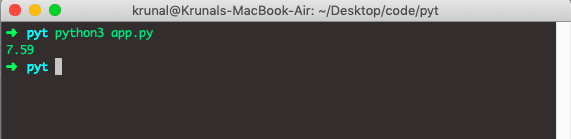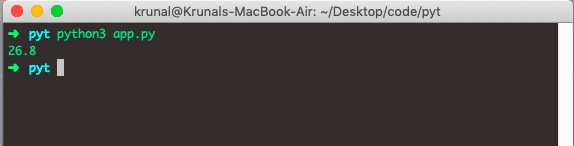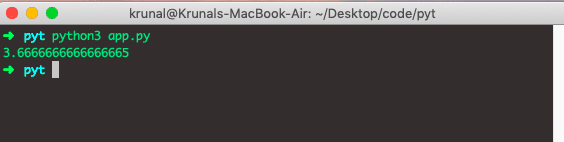# Python mean: How to Calculate Average in Python

The Statistics module in Python provides functions for calculating numeric (Real-valued) data statistics. The arithmetic mean is a sum of data divided by the number of data points. It measures the central location of data in a set of values that vary in range.

Python 3 provides the statistics module with handy functions like mean, medianmode, etc.

## Python mean

The mean() is a built-in Python statistics function used to calculate the average of numbers and lists. The mean() function accepts data as an argument and returns the mean of the data.

To use the mean() method in the Python program, import the Python statistics module, and then we can use the mean function to return the mean of the given list.

Let’s see some examples of the Statistics module.

```# app.py

import statistics

data = [11, 21, 11, 19, 46, 21, 19, 29, 21, 18, 3, 11, 11]

x = statistics.mean(data)
print(x)

y = statistics.median(data)
print(y)

z = statistics.mode(data)
print(z)

a = statistics.stdev(data)
print(a)

b = statistics.variance(data)
print(b)
```

See the following output.

```➜  pyt python3 app.py
18.53846153846154
19
11
10.611435534486562
112.6025641025641
➜  pyt
```

In the above code example, we have used Mean, mode, median, variance, stddev functions.

## Python average of a list

To calculate a mean or average of the list in Python,

1. Using statistics.mean() function.
2. Use the sum() and len() functions.
3. Using Python numpy.mean().

The formula to calculate the average is achieved by calculating the sum of the numbers in the list divided by a count of numbers in the list.

```# app.py

import statistics

spiList = [5.55, 5.72, 7.3, 7.75, 8.4, 9, 8.8, 8.2]
print(statistics.mean(spiList))
```

See the following output.In the above example, we have eight data points and use statistics.mean() function, we calculated the mean of the list.

## Using For loop to calculate the mean

In the following code example, we have initialized the variable sumOfNumbers to 0 and used a for loop.

The for loop will loop through the elements present in the list, and each number is added and saved inside the sumOfNumbers variable.

The average is calculated using the sumOfNumbers divided by the count of the numbers in the list using the len() built-in function.

```# app.py

def averageOfList(num):
sumOfNumbers = 0
for t in num:
sumOfNumbers = sumOfNumbers + t

avg = sumOfNumbers / len(num)
return avg

print("The average of List is", averageOfList([19, 21, 46, 11, 18]))```

#### Output

```python3 app.py
The average of List is 23.0```

In the above code, we use the for loop to the sum of all items and then divide that sum by several items to get the average in Python.

## Using sum() and len() functions

Python sum() is a built-in function that returns the sum of all list elements. Likewise, the len() function gives the number of items in the list. We will use the combination of these two built-in functions to get the mean of the list.

```# app.py

def averageOfList(numOfList):
avg = sum(numOfList) / len(numOfList)
return avg

print("The average of List is", round(averageOfList([19, 21, 46, 11, 18]), 2))```

#### Output

```python3 app.py
The average of List is 23.0```

## Using numpy.mean() function

NumPy.mean() function returns the average of the array elements. The average is taken over the flattened array by default; otherwise over the specified axis.

Numpy library is a commonly used library to work on large multi-dimensional arrays. It also has an extensive collection of mathematical functions on arrays to perform various tasks.

One important thing to note here is that the mean() function will give us the average for the list provided.

See the below code.

```# app.py

from numpy import mean
number_list = [19, 21, 46, 11, 18]
avg = mean(number_list)
print("The average of List is ", round(avg, 2))
```

#### Output

```python3 app.py
The average of List is 23.0```

## More Examples

First, we will import the statistics module and then call the mean() function to get the mean of data.

```# app.py

import statistics

data = [21, 19, 18, 46, 30]
print(statistics.mean(data))```

See the below output.## Calculating the Mean of a tuple in Python.

To find the mean of a tuple in Python, use the statistics.mean() method is the same as finding the list’s mean. We have to pass the tuple as a parameter.

Let’s calculate the mean of the tuple using the following code.

```# app.py

import statistics

tupleA = (1, 9, 2, 1, 1, 8)
print(statistics.mean(tupleA))```

The output of the above code is the following.It will work the same as a list. It merely returns the Mean of the numbers inside the tuple.

## Calculating the Mean of Dictionary in Python

To calculate the mean of the Dictionary, we can use statistics.mean() method. In Dictionary, the mean function only counts the keys as numbers and returns the mean of that Dictionary based on the dictionary keys.

```# app.py

import statistics

dictA = {1: 19, 2:21, 3:18, 4:46, 5:30}
print(statistics.mean(dictA))
```

See the below output.## Calculating a mean of a tuple of a negative set of integers

We use statistics to find the mean of a tuple of the negative set.mean() method. We need to pass the negative tuple as a parameter to the mean() function, and in return, we will get the output.

Let’s see the tuple of negative integers.

```# app.py

import statistics

data = (-11, -21, -18, -19, -46)
print(statistics.mean(data))

```

See the following output.

```➜  pyt python3 app.py
-23
➜  pyt
```

Let’s take an example of the tuple of a mixed range of numbers.

```# app.py

import statistics

data = (11, 21, -18, 19, -46)
print(statistics.mean(data))
```

See the following output.

```➜  pyt python3 app.py
-2.6
➜  pyt
```

## Calculating the mean of a list of a negative set of integers

To calculate the mean of the list in Python, use statistics.mean() method. We will pass the list of the negative set to the mean() method, and in the output, we will calculate the mean.

Let’s take a list of negative integers and apply the mean() function.

```# app.py

import statistics

data = [-11, -21, -18, -19, -46]
print(statistics.mean(data))

```

See the following output.

```➜  pyt python3 app.py
-23
➜  pyt
```

Let’s take an example of the list of a mixed range of numbers.

```# app.py
import statistics

data = [11, 21, -18, -19, 46]
print(statistics.mean(data))
```

See the output.

```➜  pyt python3 app.py
8.2
➜  pyt
```

## Calculating the mean of the list of fractions

To calculate the mean of the list of fractions, use statistics.mean() method. First, we have to import the statistics and fractions module and then create a fraction of numbers, and in the output, we will get the mean values.

```# app.py

import statistics
from fractions import Fraction as fr

data = [fr(1, 2), fr(44, 12), fr(10, 3), fr(2, 3)]

print(statistics.mean(data))
```

See the following output.

```➜  pyt python3 app.py
49/24
➜  pyt

```

## TypeError in Python

Let’s take string keys in the Python dictionary and get the mean of the string values. It will get the error because we can not find the string’s mean value.

See the following code.

```# app.py

import statistics

data = {"a": 11, "b": 21, "c": 19,
"d": 29, "e": 18, "f": 46}

print(statistics.mean(data))

```

See the following output.

```➜  pyt python3 app.py
Traceback (most recent call last):
File "app.py", line 6, in
print(statistics.mean(data))
File "/Library/Frameworks/Python.framework/Versions/3.6/lib/python3.6/statistics.py", line 312, in mean
T, total, count = _sum(data)
File "/Library/Frameworks/Python.framework/Versions/3.6/lib/python3.6/statistics.py", line 148, in _sum
for n,d in map(_exact_ratio, values):
File "/Library/Frameworks/Python.framework/Versions/3.6/lib/python3.6/statistics.py", line 230, in _exact_ratio
raise TypeError(msg.format(type(x).__name__))
TypeError: can't convert type 'str' to numerator/denominator
➜  pyt
```

## Conclusion

We can use the following ways to find the average or mean of the List in Python.

1. Using the statistics.mean() method.
2. Using Python sum() and len() method.
3. Using Numpy.mean() function.
4. Using for loop and len() method.

That’s it.

### 2 thoughts on “Python mean: How to Calculate Average in Python”

1.many thanks a good deal this website is actually professional and simple

2.